# Understanding PCA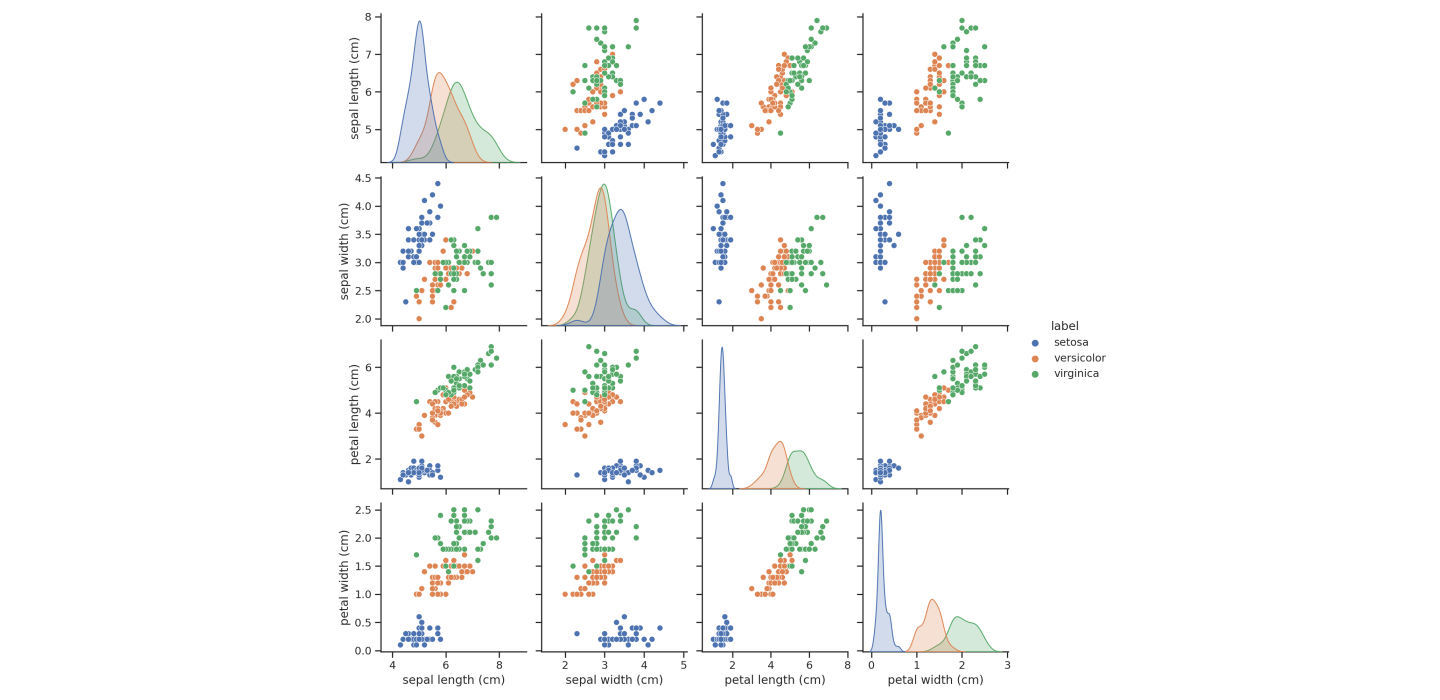The ability to look at the data with your eyes is one of the most under-appreciated things in machine learning. Before any machine learning is done, visualizing the data can reveal a lot of patterns. Exploring them can pay huge dividends later, as we can get a good intuition about what algorithm to use, which features to omit, etc.

However, there is a big issue: humans cannot see in dimensions larger than three. So, for real-life datasets, we have to perform certain tricks to benefit from the insight that visualization can provide.

The Principal Component Analysis (PCA) is one of the most basic and useful methods for this purpose. PCA linearly transforms the data into a space that highlights the importance of each new feature, thus allowing us to prune the ones that doesn't reveal much.

First, we will get an intuitive understanding of PCA, and then we proceed with a more detailed mathematical treatment.

## The main idea behind PCA

To get a good grip on what PCA does, we shall see how it performs on a simple dataset. Suppose that our data is stored in the matrix

$X \in \mathbb{R}^{n \times d},$

where $\textstyle n$ represents the features and $\textstyle d$ represents the number of samples. For simplicity, we shall assume that $n = 2$ so we can visualize what is happening. However, all that will be said holds for the general case.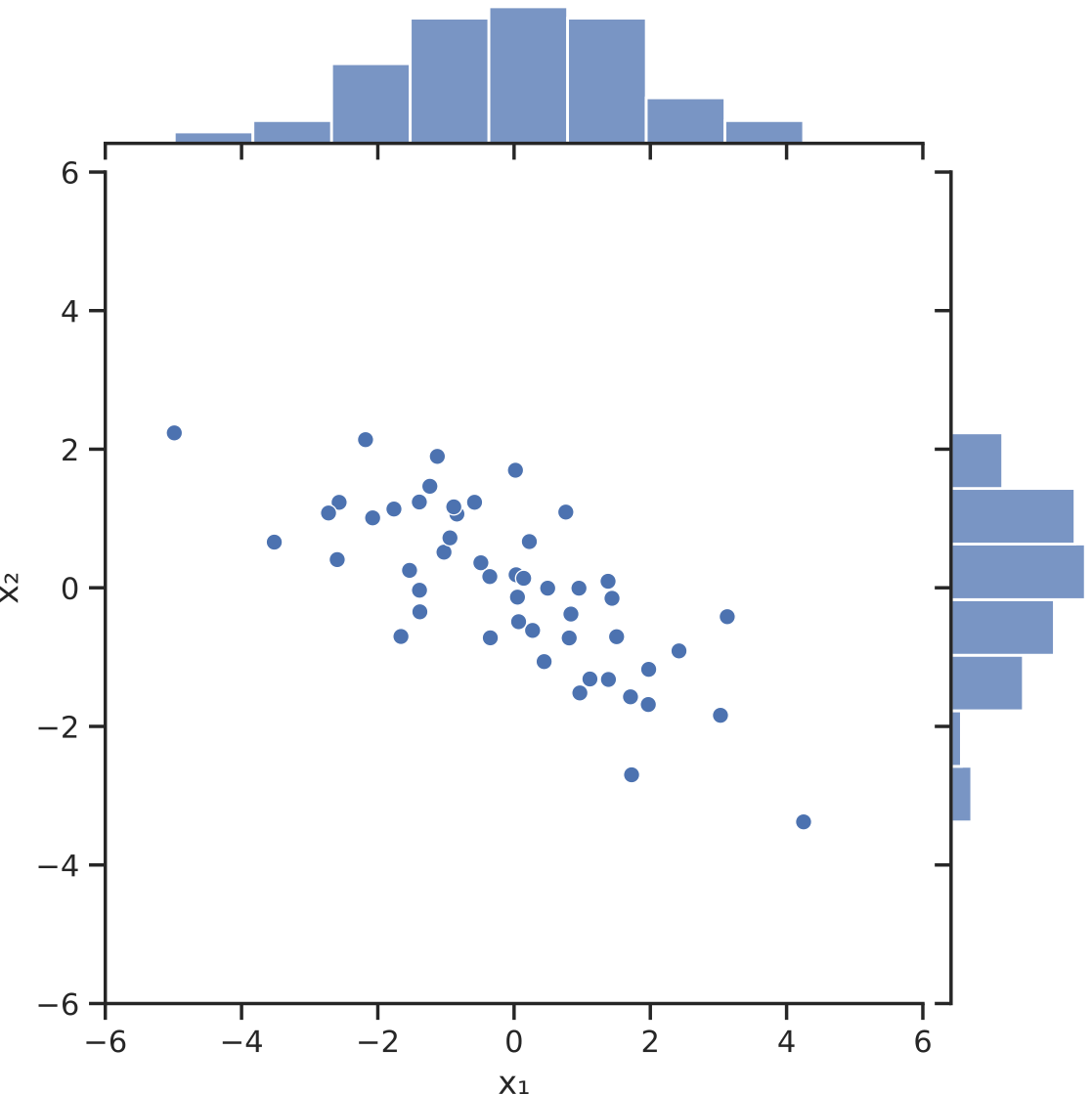What we can observe right away is that the features $x_1$ and $x_2$ are perhaps not the best descriptors of our data. The distribution seems to be stretched out across both variables, although the shape suggests that the data can be simplified from a particular viewpoint. The features seem correlated: if $x_1$ is small, then $x_2$ is large. If $x_1$ is large, $x_2$ is small. This means that the variables contain redundant information. Redundancy is suboptimal when we have hundreds of features. If we could reshape the data to a form where the variables are uncorrelated and ordered according to importance, we would be able to discard the ones that are not that expressive.

We can formulate this process in the language of linear algebra. To decorrelate the data, we are looking to diagonalize the covariance matrix of $\textstyle X$. Since the empirical covariance matrix

$\text{Cov}[X] = \frac{1}{d}(X - \overline{X})(X - \overline{X})^T, \quad \overline{X} = \frac{1}{d} \sum_{k=1}^{d} X_k$

is symmetric, the spectral decomposition theorem guarantees as an orthogonal matrix U such that

$U^T \text{Cov}[X] U = \begin{bmatrix} \lambda_1 & 0 & \dots & 0 \\ 0 & \lambda_2 & \dots & 0 \\ \vdots & \vdots & \ddots & \vdots \\ 0 & 0 & \dots & \lambda_n \end{bmatrix}, \quad \lambda_1 \geq \dots \geq \lambda_n.$

(Note that without the loss of generality, we can assume that the elements in the diagonal are decreasing.)

So, we have

\begin{align*} U^T \text{Cov}[X] U &= U^T \text{Cov}[X - \overline{X}] U \\ &= \text{Cov}[U^T(X - \overline{X})]. \end{align*}

If we define the transform $U^T(X - \overline{X})$, the variable $\textstyle Y$ represents a view of the data with uncorrelated features. This is the essence of principal component analysis. Each feature of $\textstyle Y$ is a linear combination of the features of $\textstyle X$. $\textstyle Y$ is called the principal component vector of $\textstyle X$, while its features are called principal components.

Applied to our simple dataset, this is the result.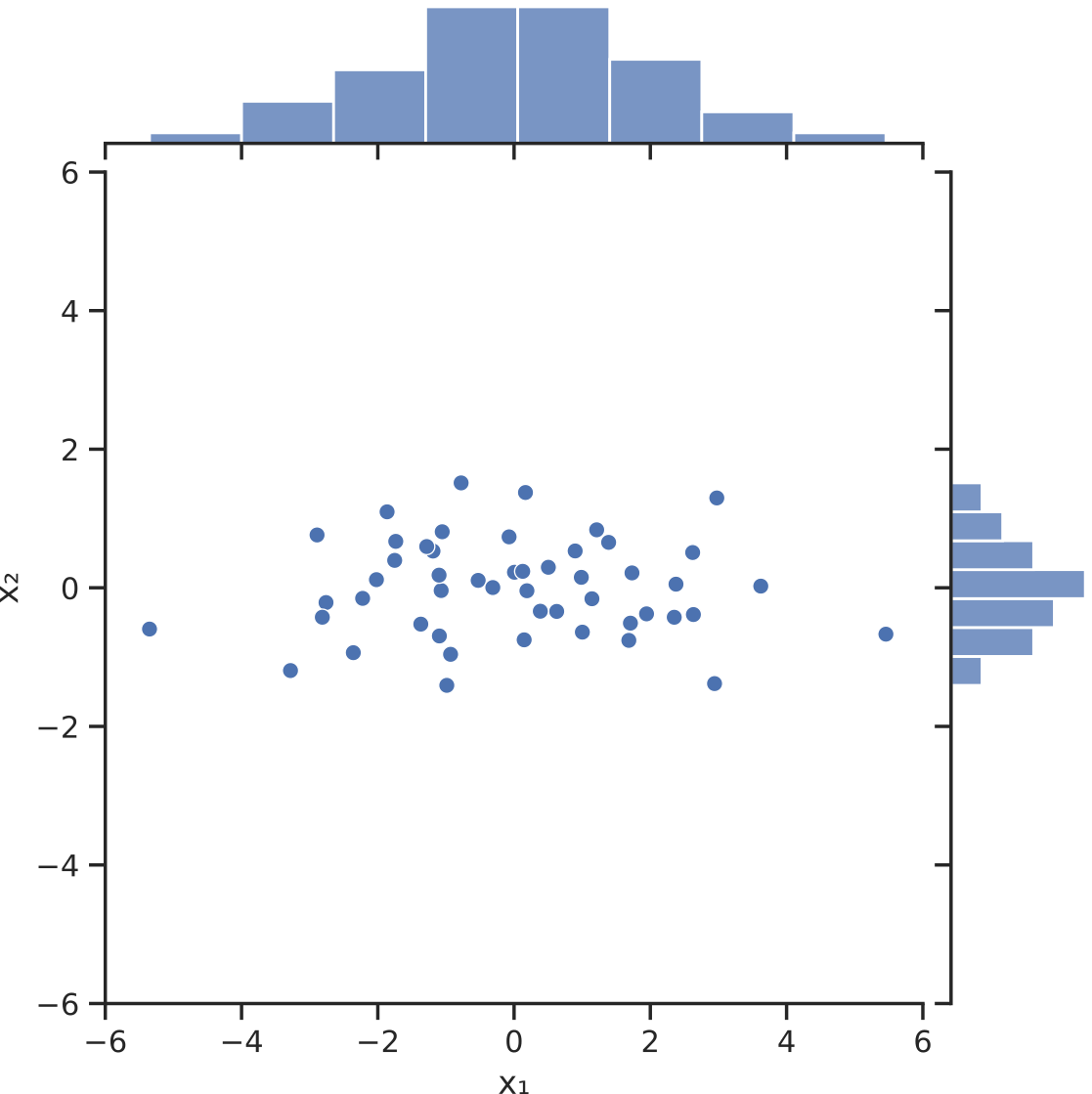## The principal components maximize variance

Besides the uncorrelated nature $\textstyle Y$, there is one thing that makes its features unique: each principal component is maximizing the variance of the data when projected on it.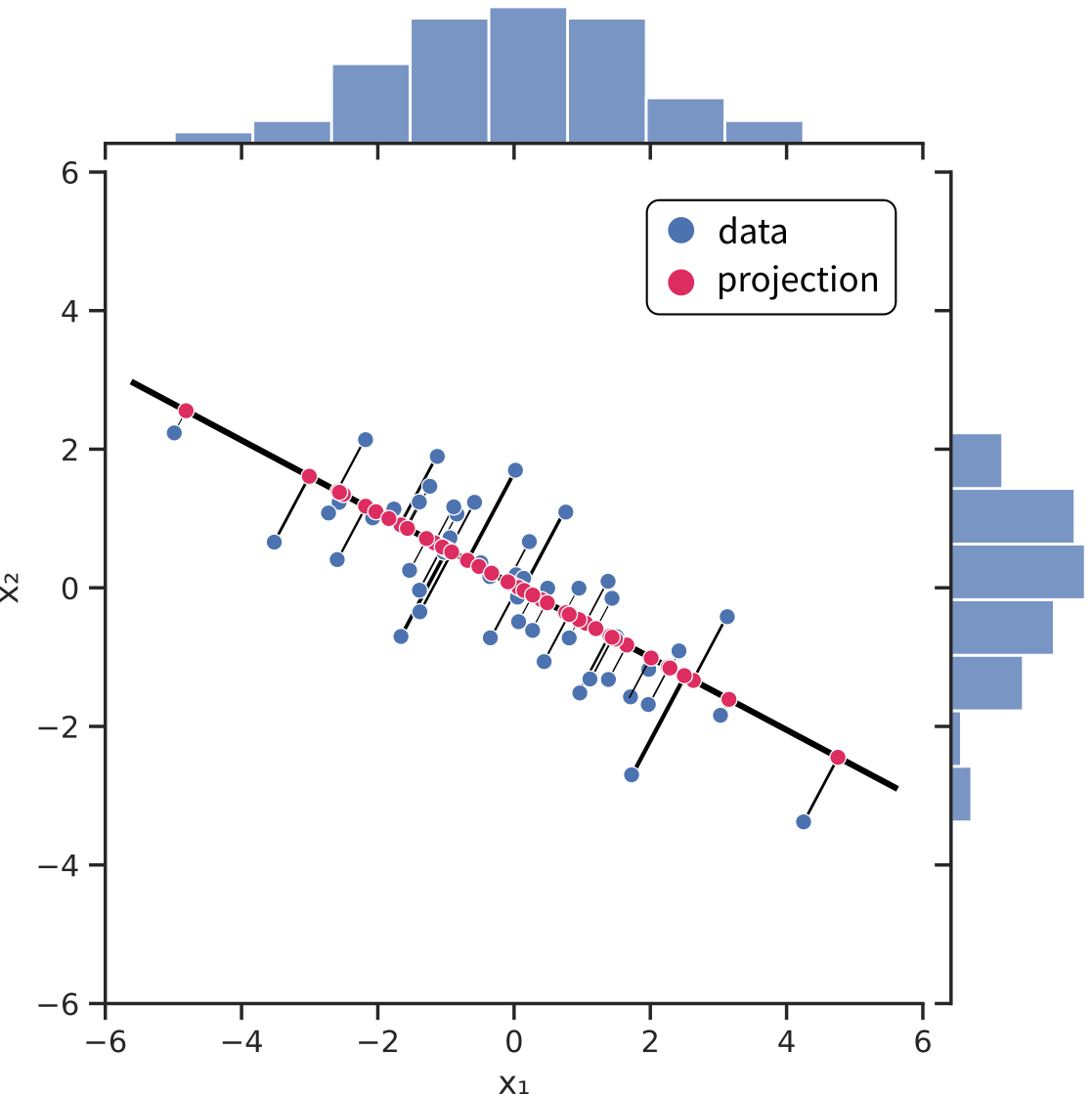PCA can be thought of as an iterative process that finds directions along which the variance of projected data is maximal.

In general, the $\textstyle k$-th principal component can be obtained by finding the unit vector that is orthogonal to the first $k-1$ principal components and maximizes the variance of the data projected to it.

## Dimensionality reduction with PCA

Now that we understand how PCA works, we should look into how it works on real datasets. Besides eliminating redundancies in the features, it can also be used to prune those that don't convey a lot of information. Recall that the covariance matrix of $U^T(X - \overline{X})$ is diagonal, and the features' variance are ordered in decreasing order:

\begin{align*} \text{Cov}[Y] = \begin{bmatrix} \text{Cov}[\mathbf{y}_1] & 0 & \dots & 0 \\ 0 & \text{Cov}[\mathbf{y}_2] & \dots & 0 \\ \vdots & \vdots & \ddots & \vdots \\ 0 & 0 & \dots & \text{Cov}[\mathbf{y}_n] \end{bmatrix}, \quad \text{Cov}[\mathbf{y}_1] \geq \dots \geq \text{Cov}[\mathbf{y}_n]. \end{align*}

The explained variance of $\mathbf{y}_k$ is defined by the ratio

$\frac{\text{Cov}[\mathbf{y}_k]}{\sum_{i=1}^{n} \text{Cov}[\mathbf{y}_i]} \in [0, 1]$

which can be thought of as the percentage of "all the variance" in the data. Since the variances are decreasing, the most meaningful features come first. This means that if the cumulative explained variance

$\sum_{j=1}^{k} \frac{\text{Cov}[\mathbf{y}_j]}{\sum_{i=1}^{n} \text{Cov}[\mathbf{y}_i]}$

is large enough for some k, say around 95%, principal components after $\textstyle k$ can be dropped without significant information loss. To see how it performs on real data, we will see how PCA performs on the famous Iris dataset! This dataset consists of four features (sepal length, sepal width, petal length, petal width) from three different kinds of irises Setosa, Versicolour, and Virginica. This is how the dataset looks.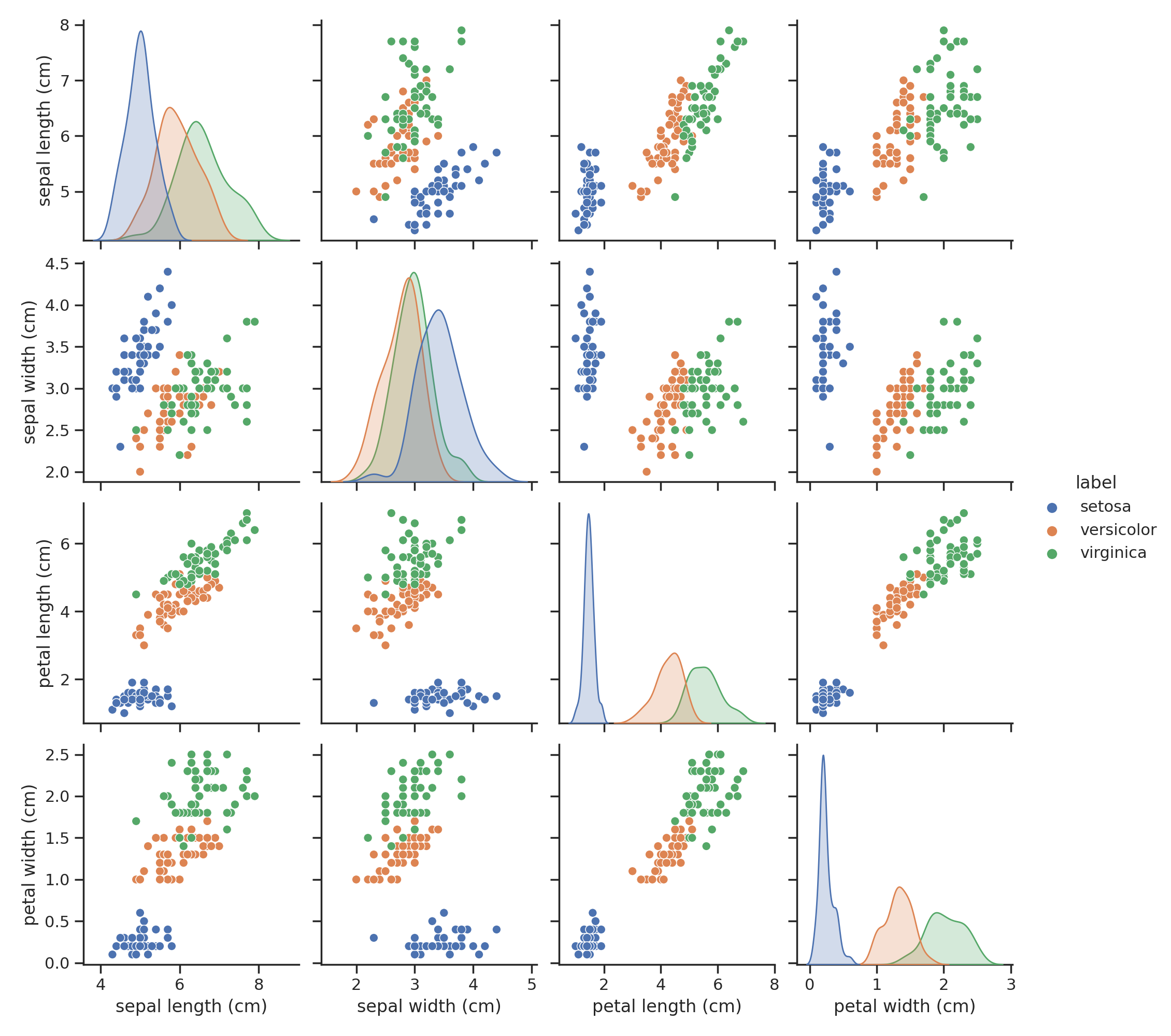Upon inspection, we can see that some combination of features separates the data well, some don't. Their order definitely doesn't signify their importance. By calculating the covariance matrix, we can also notice that they seem to be correlated:

[[ 0.68112222 -0.04215111 1.26582 0.51282889]
[-0.04215111 0.18871289 -0.32745867 -0.12082844]
[ 1.26582 -0.32745867 3.09550267 1.286972 ]
[ 0.51282889 -0.12082844 1.286972 0.57713289]]


Let's apply PCA to see what it does with our dataset!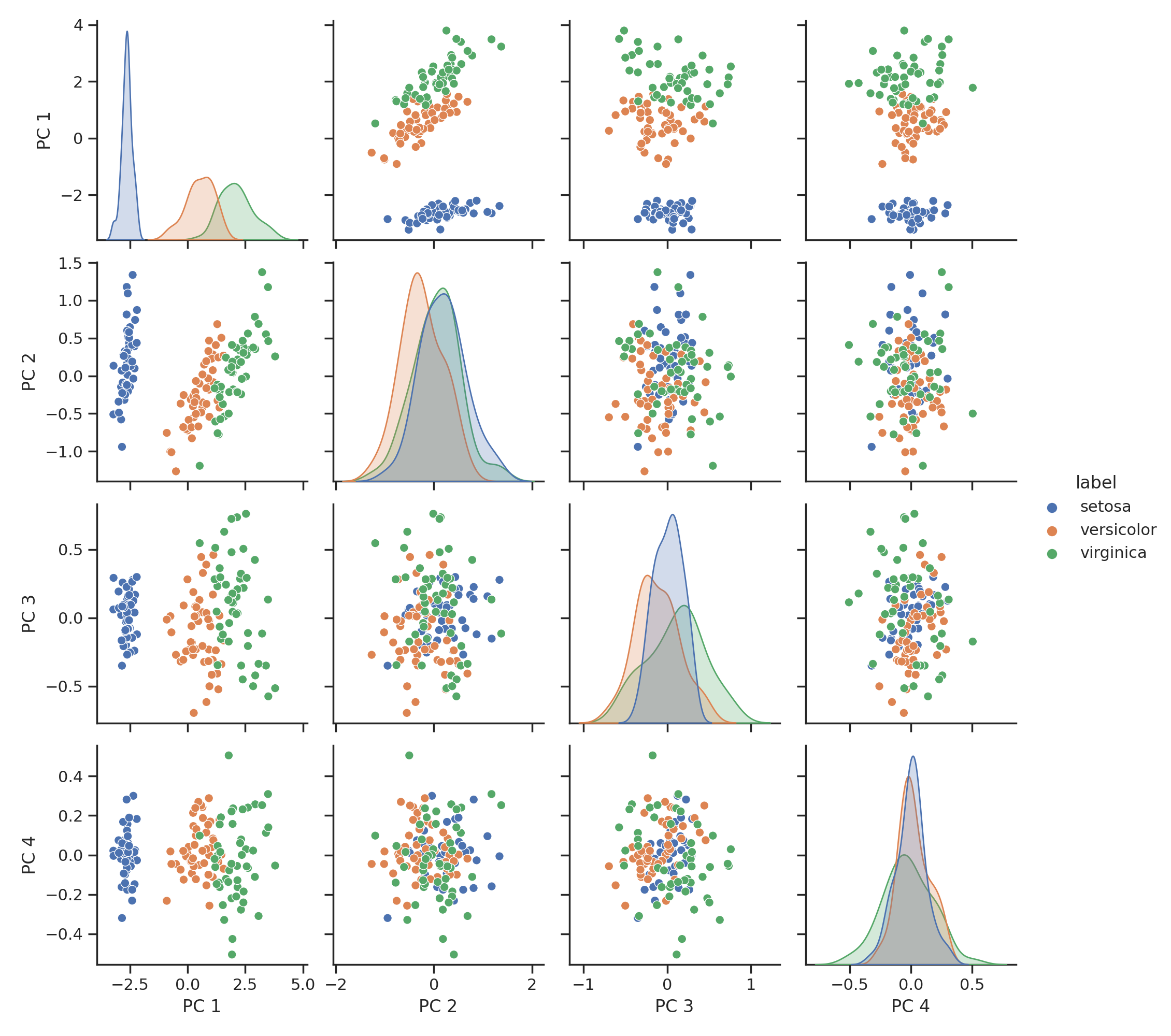The first two principal component almost completely describe the dataset, while the 3rd and 4th can be omitted without noticeable loss of information. The covariance matrix also looks much better. It is essentially diagonal, so the features are not correlated with each other.

[[ 1.57502004e+02 -3.33667355e-15 2.52281147e-15 5.42949940e-16]
[-3.33667355e-15 9.03948536e+00 1.46458764e-15 1.37986097e-16]
[ 2.52281147e-15 1.46458764e-15 2.91330388e+00 1.97218052e-17]
[ 5.42949940e-16 1.37986097e-16 1.97218052e-17 8.87857213e-01]]


## What the PCA doesn't do

Although PCA is frequently used for feature engineering, there are limits on what it can do. For instance, if the dataset is thinly stretched out and the separating margin is small between them (like the example below), PCA won't provide a representation where the difference between classes is sharper.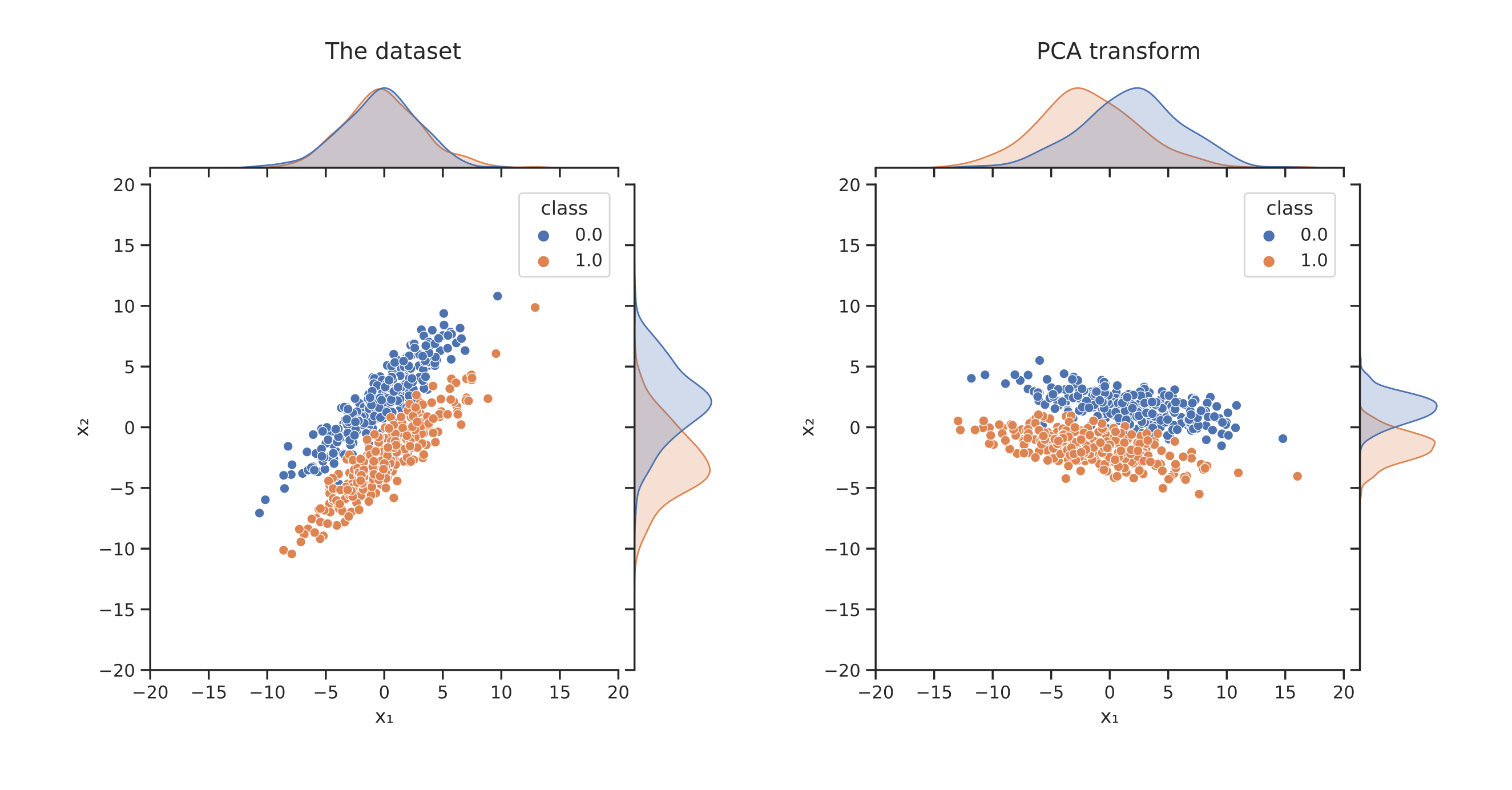The reason is that an orthogonal transformation gives the principal component vectors, and orthogonal transformations preserve distance. So, this doesn't stretch the space along any features. This is an advantage and a disadvantage at the same time. In two dimensions, an orthogonal transformation is just a composition of rotations and reflections.